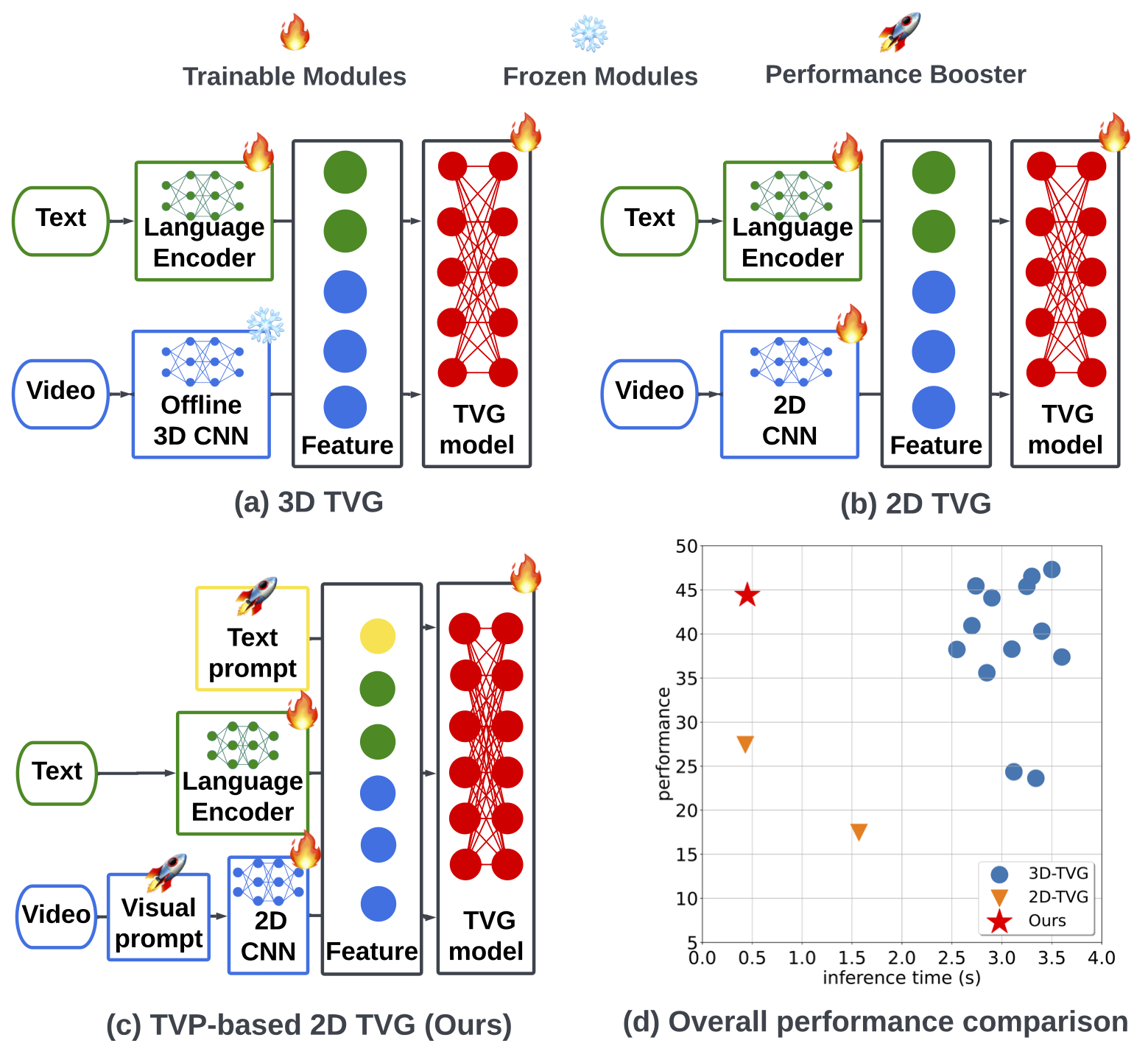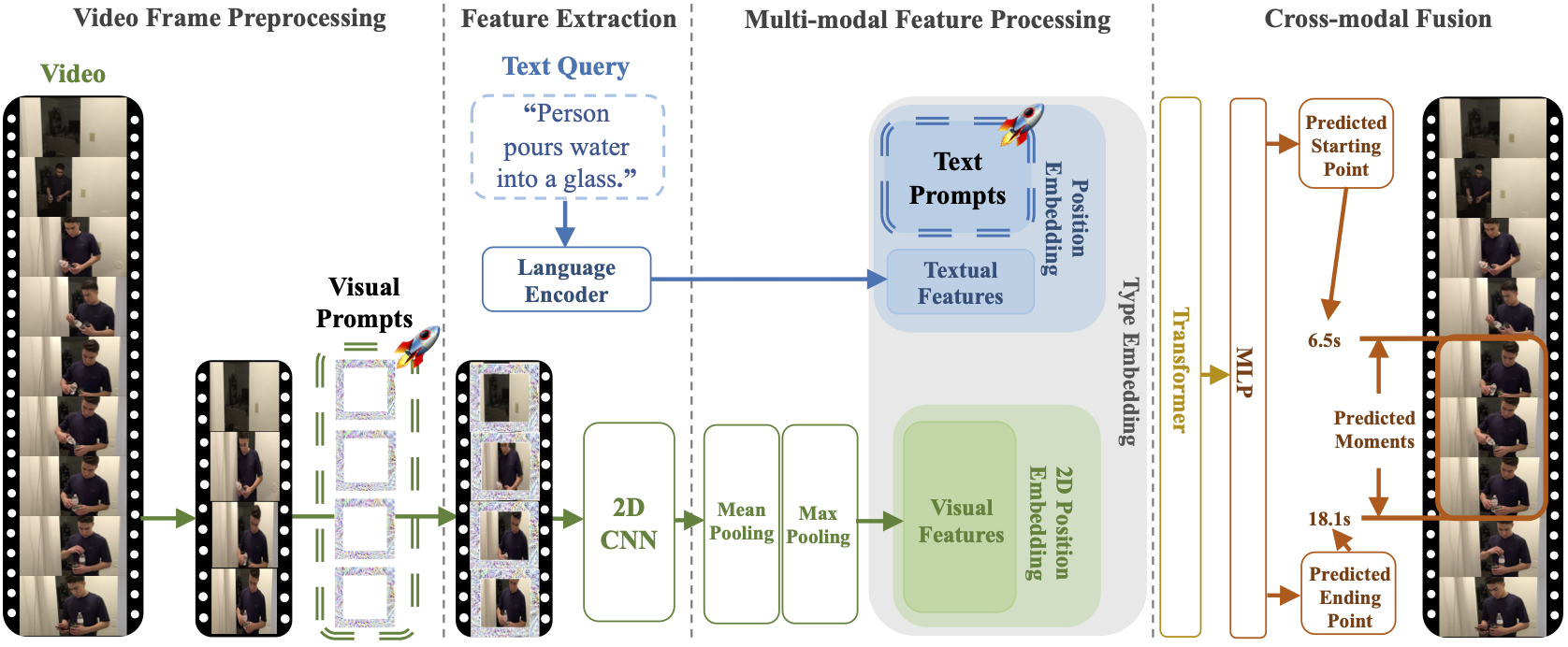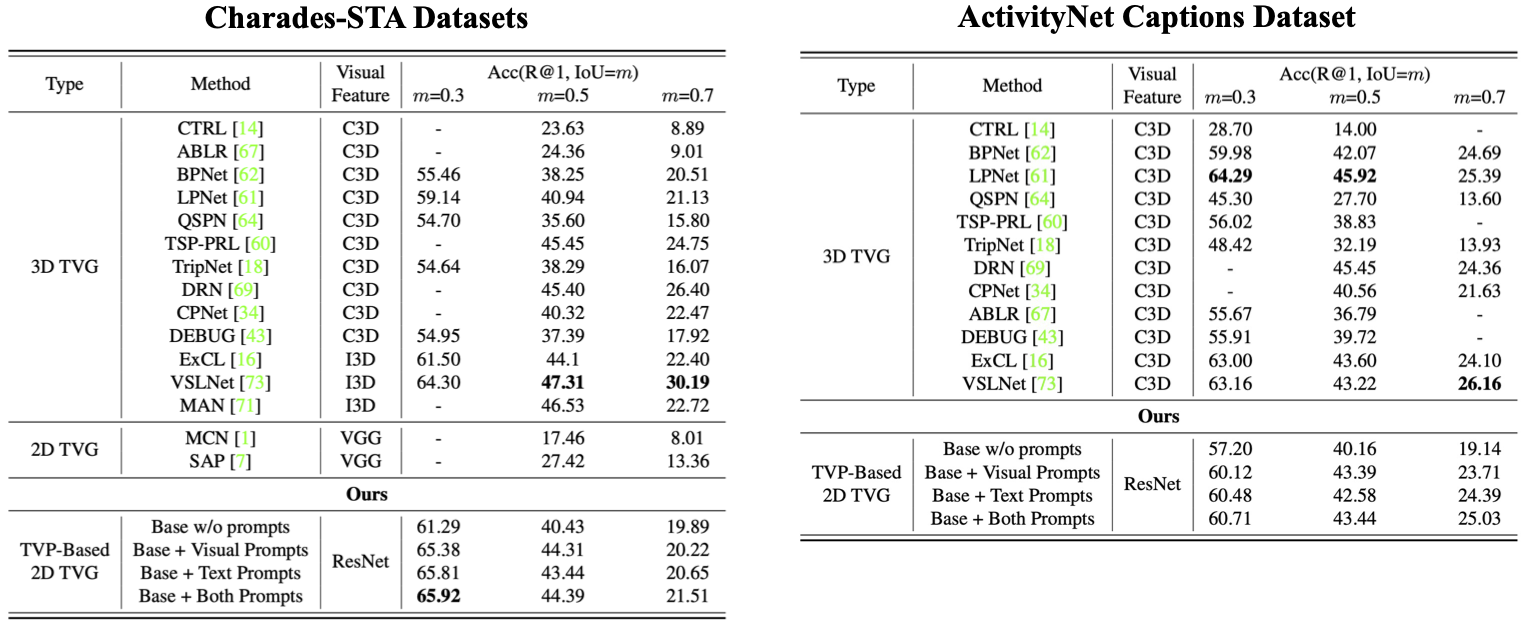How to advance 2D TVG methods so as to achieve comparable results to 3D TVG methods?

## Abstract

In this paper, we study the problem of temporal video grounding (TVG), which aims to predict the starting/ending time points of moments described by a text sentence within a long untrimmed video. Benefiting from fine-grained 3D visual features, the TVG techniques have achieved remarkable progress in recent years. However, the high complexity of 3D convolutional neural networks (CNNs) makes extracting dense 3D visual features time-consuming, which calls for intensive memory and computing resources. Towards efficient TVG, we propose a novel text-visual prompting (TVP) framework, which incorporates optimized perturbation patterns (that we call ‘prompts’) into both visual inputs and textual features of a TVG model. In sharp contrast to 3D CNNs, we show that TVP allows us to effectively co-train vision encoder and language encoder in a 2D TVG model and improves the performance of crossmodal feature fusion using only low-complexity sparse 2D visual features. Further, we propose a Temporal-Distance IoU (TDIoU) loss for efficient learning of TVG. Experiments on two benchmark datasets, Charades-STA and Ac- tivityNet Captions datasets, empirically show that the pro- posed TVP significantly boosts the performance of 2D TVG (e.g., 9.79% improvement on Charades-STA and 30.77% improvement on ActivityNet Captions) and achieves 5× inference acceleration over TVG using 3D visual features.

## Our Proposal: TVP FrameworkOverview of our proposed TVP (text-visual prompting) framework for 2D TVG (temporal video grounding).

Inspired by the success of transformers in vision-language tasks, we choose ClipBERT  as the base model for 2D TVG. Extended from ClipBERT, the input of our regression-based TVG model would be describable sentences and uniformly sampled frames of one untrimmed video as shown in figure above. Then, the predicted starting and ending time points of the target video clip would be model outputs.

There are four phases of our proposed TVP framework:

1. Video frame preprocessing: We obtain sparsely-sampled frames $$\mathbf{v}_\mathrm{sam}$$ from one input untrimmed video $$\mathbf{v}$$, and apply universal frame-aware visual prompts $$\boldsymbol{\delta}_{\mathrm{vp}}$$ on top of frames at the padding location.

2. Feature extraction: 2D vision encoder (first 5 ConvBlock of ResNet-50) $$g_\mathrm{vid}$$ and language encoder (a trainable word embedding layer) $$g_\mathrm{tex}$$ would extract features from the prompted frames $$\mathbf{v}^{\prime}_\mathrm{sam}$$ and textual inputs $$\mathbf{s}$$, respectively.

3. Multimodal feature processing: Following the setting of Pixel-BERT , the 2D visual features $$\mathbf{Q}_\mathrm{vid}$$ are downsampled spatially by a $$2\times2$$ max-pooling layer and fused temporally by a mean-pooling layer. Then, text prompts $$\boldsymbol{\delta}_{\mathrm{tp}}$$ are integrated into textual features $$\mathbf{Q}_\mathrm{tex}$$. In addition, trainable 2D visual position embeddings $$\mathbf{M}_\mathrm{2D}$$ and textual position embeddings $$\mathbf{M}_\mathrm{pos}$$ are applied to the processed 2D visual features $$\mathbf{Q}^{\prime}_{\mathrm{vid}}$$ and prompted textual features $$\mathbf{Q}^{\prime}_\mathrm{tex}$$, respectively [1, 3]. Afterwards, the processed and position-encoded 2D visual features $$\mathbf{Q}^{\prime\prime}_{\mathrm{vid}}$$ are flattened and integrated into prompted and position-encoded textual features $$\mathbf{Q}^{\prime\prime}_\mathrm{tex}$$. Moreover, type embeddings $$\mathbf{M}_\mathrm{type}$$ would be added to the integrated multimodal features $$\mathbf{Q}_{\mathrm{all}}$$ to indicate the source type of features.

4. Crossmodal fusion: A 12-layer transformer  is utilized for crossmodal fusion on $$\mathbf{Q}_{\mathrm{all}}$$, and then multilayer perceptron (MLP) ending with sigmoid function is used as the prediction head to process the last-layer \underline{c}ross\underline{m}odal representation $$\mathbf{Q}_\mathrm{CM}$$ of the transformer for generating the predicted starting/ending time points $$(\hat{t}_\mathrm{sta}, \hat{t}_\mathrm{sta})$$ of the target moments described by the text query input.

## Performance Comparison

Our method yields substantial accuracy improvement on multiple datasets.Performance comparison of different thresholds m on the Charades-STA and ActivityNet Captions Datasets.

## Citation

@article{zhang2023text,
title={Text-visual prompting for efficient 2d temporal video grounding},
author={Zhang, Yimeng and Chen, Xin and Jia, Jinghan and Liu, Sijia and Ding, Ke},
journal={arXiv preprint arXiv:2303.04995},
year={2023}
}


 Lei et al. (2021). Less is More: ClipBERT for Video-and-Language Learning via Sparse Sampling.

 Huang et al. (2020). Pixel-BERT: Aligning Image Pixels with Text by Deep Multi-Modal Transformers.

 Devlin et al. (2018). BERT: Pre-training of Deep Bidirectional Transformers for Language Understanding.